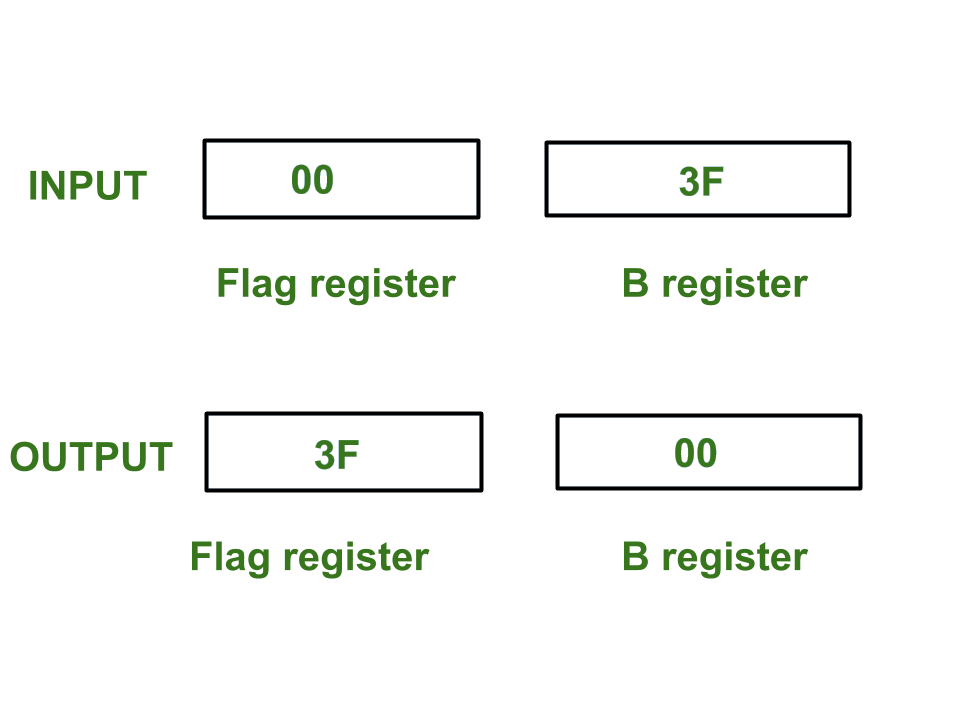Open in App
Not now

# 8085 program to access and exchange the content of Flag register with register B

• Last Updated : 17 Jan, 2022

Problem – Write an assembly language program in 8085 microprocessor to access Flag register and exchange the content of flag register F with register B.

Example –Assumptions – Initial values of flag register, register B and stack pointer are is 00, 3F, and 3FFF respectively.

PSW stands for PROGRAM STATUS WORD. PSW combines accumulator A and flag register F.

Algorithm –

1. Push the value of PSW in-memory stack with the help of PUSH instruction

2. Pop the value of the Flag register and store it in register H with help of POP instruction

3. Move the value of register L in register C  [ H -> ACCUMULATOR VALUE, L-> FLAG REGISTER VALUE]

4. Move the value of register B in register L

5. Move the value of register C in register B

6. Push the value of register H in-memory stack with the help of PUSH instruction

7. Pop the value of PSW from the memory stack using POP instruction

Program –

```MVI B , 3FH
PUSH PSW
POP H
MOV C , L
MOV L , B
MOV B , C
PUSH H
POP PSW
HLT```

Explanation – Registers used A, B, C, H, F

1. PUSH PSW instruction performs the following task:

```    SP <- SP - 1
M[SP] <- A
SP <- SP - 1
M[SP] <- F```
1. POP H instruction performs the following task:

```    H <- M[SP]
SP <- SP + 1```
1. MOV C, L – moves the value of L in register C as we know that H contains the accumulator value and L contains flag register value

2. MOV L, B – moves the value of B in register L, hence L is updated

3. MOV B, C – moves the value of C in register B, hence B is updated

4. PUSH H performs the following task:

```    SP <- SP - 1
M[SP] <- H```
1. POP PSW performs the following task:

```
F <- M[SP]
SP <- SP + 1
A <- M[SP]
SP <- SP + 1```
1. HLT – stops executing the program and halts any further execution

My Personal Notes arrow_drop_up
Related Articles